﻿ A New Method for Solving Fully Fuzzy Linear Programming with LR- type Fuzzy Numbers

### A New Method for Solving Fully Fuzzy Linear Programming with LR- type Fuzzy Numbers

M. M. Shamooshaki, A. Hosseinzadeh, S. A. EdalatpanahOPEN ACCESSPEER-REVIEWED

## A New Method for Solving Fully Fuzzy Linear Programming with LR- type Fuzzy Numbers

M. M. Shamooshaki1, A. Hosseinzadeh1, S. A. Edalatpanah2,1Department of Mathematics, Comprehensive Imam Hossein University, Tehran, Iran

2Department of Applied Mathematics, Tonekabon Branch, Islamic Azad University, Tonekabon, Iran

### Abstract

One of the most practicable subjects in recent studies is based on LR fuzzy number, which was defined and used by Dubois and Prade with some useful and easy approximation arithmetic operators on them. In this paper, a new method is proposed to find the fuzzy optimal solution of fully fuzzy Linear Programming (FFLP) problems with equality constraints. We use also LR-type fuzzy numbers and our model covers a wide class of fuzzy numbers, such as triangular fuzzy numbers. To illustrate our method, a numerical example is solved.

• Shamooshaki, M. M., A. Hosseinzadeh, and S. A. Edalatpanah. "A New Method for Solving Fully Fuzzy Linear Programming with LR- type Fuzzy Numbers." International Journal of Data Envelopment Analysis and *Operations Research* 1.3 (2014): 53-55.
• Shamooshaki, M. M. , Hosseinzadeh, A. , & Edalatpanah, S. A. (2014). A New Method for Solving Fully Fuzzy Linear Programming with LR- type Fuzzy Numbers. International Journal of Data Envelopment Analysis and *Operations Research*, 1(3), 53-55.
• Shamooshaki, M. M., A. Hosseinzadeh, and S. A. Edalatpanah. "A New Method for Solving Fully Fuzzy Linear Programming with LR- type Fuzzy Numbers." International Journal of Data Envelopment Analysis and *Operations Research* 1, no. 3 (2014): 53-55.

 Import into BibTeX Import into EndNote Import into RefMan Import into RefWorks

### 1. Introduction

Linear Programming (LP) is one of the most important fields of operation research that, given its efficiency in different areas, has developed during recent decades. Linear programming is the process of taking various linear inequalities relating to some situation, and finding the "best" value obtainable under those conditions. Linear programming is in two forms: classical linear programming and fuzzy linear programming (FLP) in which the variables are assessed in a fuzzy manner.

In conventional approaches, the value of parameters in LP models must be well defined and precise. However, in real world environment, this is not a realistic assumption.

In real world applications, certainty, reliability and precision of data is often illusory. The optimal solution of an LP only depends on a limited number of constraints; therefore much of the collected information has little impact on the solution. It is useful to consider the knowledge of experts about the parameters as fuzzy data.In such a situation the parameters of linear programming problems may be represented as fuzzy numbers (FLP). The FLP problems in which all the parameters as well as the variables are represented by fuzzy numbers are known as fully fuzzy linear programming (FFLP) problems. There are a lot of methods for solving FFLP problems. A max-min operator for converting a fuzzy decision making problem to its crisp equivalent was first proposed by Bellman and Zadeh (1979). The idea was adopted by several authors for solving FLP problems. Rommelfanger et al., (1989), Fang and Hu (1999), Maleki et al., (2000), Maleki (2002) and Khan et al., (2010) are studies where the objective functions, the decision variables, the technical coefficients and the constraints are fuzzy numbers respectively. Dehghan et.al (2006), proposed a fuzzy linear programming approach for finding the exact solution of fully fuzzy linear system of equations (FFLSE). Ebrahimnejad and Nasseri (2009) developed the complementary slackness theorem for solving FLP with fuzzy parameters. Lotfi et.al (2009), proposed a method to obtain the approximate solution of FFLP problems. By using their method, the solutions obtained are approximate and also it is very difficult to apply this method to find the fuzzy optimal solution of FFLP problems. Moreover Kumar et.al (2011) considered this problem and presented a new method for solving FFLP problems. However this method is limited to a certain class of fuzzy numbers, i.e. triangular fuzzy numbers and needed some corrections made by Saberi Najafi and Edalatpanah (2013).

In this paper, we propose a new method for solving FFLP problems by using LR- type fuzzy numbers,which include a wide class of FFLP problems. Moreover, our method dose not have the disadvantages of the above methods and compared with other metods is more efficient.

### 2. Preliminaries

In this section some basic definitions, arithmetic operations and ranking function are reviewed, taken from Zimmermann (1978), Dubois and Prade (1980), Kaufmann (1998), and Kumar (2011).

Definition 2.1. (Kaufmann (1998)) The characteristic function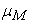of a crisp set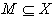assigns a value either 0 or 1 to each member in X. This function can be generalized to a function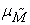such that the value assigned to the element of the universal set X fall within a specified range i.e.The assigned value indicate the membership grade of the element in the set M. The functionis called the membership function and the setDefined byfor eachis called a fuzzy set.

Definition 2.2. (Kaufmann (1998)) A fuzzy set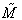, defined on the universal set of real numbers R, is said to be a fuzzy number if its membership function has the following characteristics:

1.: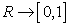is continuous.

2.for all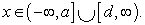3.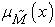strictly increasing on [a,b] and strictly decreasing on [c, d].

4.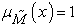for all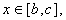where a < b < c < d.

Definition 2.3. (Zimmermann (1978)) A function, usually denoted by L or R, is a reference function of a fuzzy number if and only if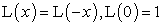and L is nonincreasing on.

Definition 2.4. (Zimmermann (1978)) A fuzzy numberis of left, right-type (LR-type), if there exist refrence functions L (for left ), R (for right), and scalers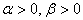with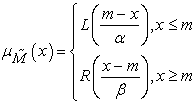The variable (m) is called value of,which is a real number and α and β are called the left and right spreads, respectively andis denoted byDefinition 2.5. (Kumar (2011))Two LR-type fuzzy numbersand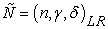are said to be equal if and only if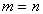and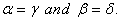Theorem 2.6. (Kumar(2011))Let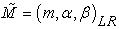,be two fuzzy numbers of LR-type. Then, we have:

(1)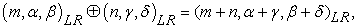(2)(3)Definition 2.7. (Dubois and Prade (1980)) A fuzzy number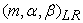is said to be non-negative fuzzy number if and only if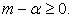Definition 2.8. (Dubois and Prade (1980)) A fuzzy numberis said to be non-negative fuzzy number if and only if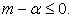Theorem 2.9. (Kumar (2011)) Let,be two fuzzy numbers of LR-type. Then, we have:

1) For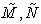are positive,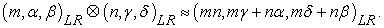2) For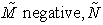positive,3) Forare negative,Definition 2.10. A ranking function (combining Zimmermann, (1978) and Kumar et.al (2011)), is a functionwhere F(R) is a set of fuzzy numbers defined on the set of real numbers, which maps each fuzzy number into a real line, where a natural order exists. Let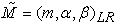be a LR-type fuzzy number, thenwhere is equal with### 3. FFLP Problem Formulation and Proposed Method for Solution

FFLP problems with m fuzzy equality constraints and n fuzzy variables can be formulated as follows:

Max (or Min)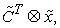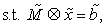(3.1)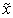is non-negetive fuzzy number.

We know that,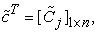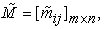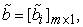and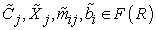are all LR-type fuzzy numbers. Thus, FFLP (3.1) can be written as:

Max(or Min)s.t.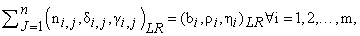(3.2)is non-negetive fuzzy number.

whereandWe assume that all coefficient fuzzy numbers are positive. The similar procedure can be performed for the other cases.

Now, the steps of the proposed method are as follows:

Step 1. By using Theorem (2.9) we first calculate multiplication of two fuzzy numbers and drawing on Definition (2.5), then by using Definition (2.10), we convert the FFLP problem (3.2) into the following linear programming problem:

Max(or Min)s. t.(3.3)

Step 2. Find the optimal solution of (3.3), say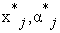and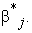Step 3. Find the fuzzy optimal solution of FFLP problem (3.2),by putting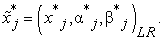Step4. Find thefuzzy optimal objective value by putting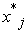in: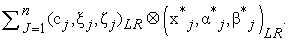Below, we solve an example which was already solved by Kumar et al. (2011) using our method with Mathematica 9 software.

Example 3.1.

Let us consider the following FFLP and solve it by the proposed method

Max(or Min)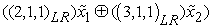s.t.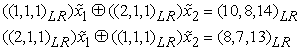and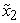are non-negative LR-type fuzzy numbers,

In view of (3.3) and definition (2.10) and, we can write this FFLP as :

Maxs.t.The optimal solution of the LP problem is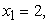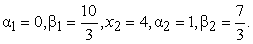Also, the optimal solution of the FFLP problem, obtained in Step 3, is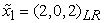and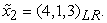Step 4 yields the optimal fuzzy objective value to be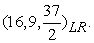This problem is also solved by Kumar et.al (2011), for wich the authors implemented the triangular fuzzy numbers. However, our method permits a great choice of auxiliary reference functions L and R, which covers the triangular fuzzy numbers as an special case. The optimal solution reached by Kumar was 17, while our optimal solution is 18.375. Our proposed method is also easy to implement compared to Dehghan et.al (2006), and Lotfi et.al(2009).

### 4. Conclusions

In this paper, a new method is developed to solve FFLP problems. This method is based on ranking function on LR -fuzzy numbers. We also solved a problem using rank function and mathematica softwar which was already solved by Kumar using TFR’s. We observed, our method is far more efficient.

### References

  Dubois and Prade, H. (1978). “Operations on fuzzy numbers”, J. Systems Sci, Vol. 9, pp. 613-626.In article CrossRef  Bellman, R. E., Zadeh, L. A. (1970). “Decision making in a fuzzy environment”, Management Science, Vol. 17, pp. 141-164.In article CrossRef  Zimmermann, H.J. (1978). “Fuzzy programming and linear programming with several objective functions”, Fuzzy Sets and Systems, Vol. 1, pp. 45-55.In article CrossRef  Rommelfanger, H., Hanuscheck, R., and Wolf, J. (1989). “Linear programming with fuzzy objective”, Fuzzy Sets and Systems, Vol. 29, pp. 31-48.In article CrossRef  Fang, S. C.,Hu, C. F. (1999). “ Linear programming with fuzzy coefficients in constraint”, Comput Math Appl, Vol. 37, pp. 63-76.In article CrossRef  Maleki, H.R., Tata, M., Mashinchi, M. (2002). “Linear programming with fuzzy Variables”, Fuzzy Sets and Systems, Vol. 109, pp. 21-33.In article CrossRef  Maleki, H.R. (2002). “Ranking functions and their applications to fuzzy linearprogramming”, Far East Journal of Mathematical Science, Vol. 4, pp. 283-301.In article  Khan, I. U., Ahmad, T., Maan. N. (2010). “ A two phase approach for solving linear programming problems by using fuzzy trapezoidal membership functions”, International Journal of Basic and Applied Sciences, vol. 6, pp. 86-95.In article  Ebrahimnejad, A., Nasseri, S.H. (2009). “Using complementary slackness property to solve linear programming with fuzzy parameters”, Fuzzy Information and Engineering, Vol. 3, pp. 233-245.In article CrossRef  Dehghan, M.,Hashemi, B., Ghatee, M. (2006). “Computational methods for solving fully fuzzy linear systems”, Appl. Math. Comput, Vol. 179, pp. 328-343.In article CrossRef  Lotfi, F.H., Allahviranloo, T., Jondabeha, M.A., Alizadeh, L. (2009). “Solving a fully fuzzy linear programming using lexicography method and fuzzy approximate solution”, Appl.Math.Modell, Vol. 33, pp. 3151-3156.In article CrossRef  Kumar, A., Kaur, J., Singh, P. (2011) “A new method for solving fully fuzzy linear programming problems”, Appl. Math. Modell, Vol. 35, pp. 817-823.In article CrossRef  Saberi Najafi, H., and S.A. Edalatpanah, (2013). “A Note on A new method for solving fully fuzzy linear programming problems”, Appl. Math. Modell, Vol. 37, pp. 7865-7867.In article CrossRef